International
Tables for
Crystallography
Volume C
Mathematical, physical and chemical tables
Edited by E. Prince

International Tables for Crystallography (2006). Vol. C, ch. 8.1, p. 682

Section 8.1.3.3. Conditioning

E. Princea and P. T. Boggsb

aNIST Center for Neutron Research, National Institute of Standards and Technology, Gaithersburg, MD 20899, USA, and bScientific Computing Division, National Institute of Standards and Technology, Gaithersburg, MD 20899, USA

8.1.3.3. Conditioning

| top | pdf |

The condition number of Z, which is defined (Subsection 8.1.1.1) as the square root of the ratio of the largest to the smallest eigenvalue of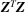, is an indicator of the effect a small change in an element of Z will have on the elements of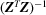and of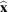. A large value of the condition number means that small errors in computing an element of Z, owing possibly to truncation or roundoff in the computer, can introduce large errors into the elements of the inverse matrix. Also, when the condition number is large, the standard uncertainties of some estimated parameters will be large. A large condition number, as defined in this way, can result from either scaling or correlation or some combination of these. To illustrate this, consider the matrices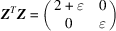and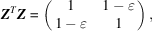whererepresents machine precision, which can be defined as the smallest number in machine representation that, when added to 1, gives a result different from 1. By the conventional definition, both of these matrices have a condition number for Z of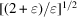. Because numbers of ordercan be perfectly well represented, however, the first one can be inverted without loss of precision, whereas an inverse for the second would be totally meaningless. It is good practice, therefore, to factor the design matrix, Z, into the form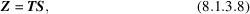where S is a p × p diagonal matrix whose elements define some kind of `natural' unit appropriate to the parameter represented in each column of Z. The ideal natural unit would be the standard uncertainty of that parameter, but this is not available until after the calculation has been completed. If correlation is not too severe, suitable values for the elements of S, of the same order of magnitude as those derived from the standard uncertainty, are the column Euclidean norms, that is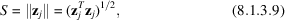where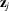denotes the jth column of Z. This scaling causes all diagonal elements of ZTZ to be equal to one, and errors in the elements of Z will have roughly equal effects.

Ill conditioning that results from correlation, as in the second example above, is more difficult to deal with. It is an indication that some linear combination of parameters, some eigenvector of the normal equations matrix, is poorly determined by the available data. Use of the QR factorization of Z to compute the Cholesky factor of ZTZ may be advantageous, in spite of the additional computation time, because better numerical stability is obtained in marginal situations. As a practical matter, however, it is important to recognize that an ill conditioned matrix is a symptom of a flaw in the model or in the experimental design (or both). Use can be made of the fact that, although determining the entire set of eigenvalues and eigenvectors of a large matrix is computationally an inherently difficult problem, a relatively simple algorithm, known as a condition estimator (Anderson et al., 1992), can produce a good approximation to the eigenvector that corresponds to the smallest eigenvalue of a nearly singular matrix. This information can be used in either or both of two ways. First, without any fundamental modification to the model or the experiment, a simple, linear transformation of the parameters so that the problem eigenvector is one of the independent parameters, followed by rescaling, can resolve the numerical difficulties in computing the estimates. A common example is the situation where a phase transition results in the doubling of a unit cell, with pairs of atoms almost but not quite related by a lattice translation. A transformation that makes the estimated parameters the sums and differences of corresponding parameters in related pairs of atoms can make a dramatic improvement in the condition number. Alternatively, the problem eigenvector can be set to some value determined from theory or from some other experiment (see Section 8.3.1), or additional data can be collected that are selected to make that combination of parameters determinate.

References

Anderson, E., Bai, Z., Bischof, C., Demmel, J., Dongarra, J., Du Croz, J., Greenbaum, A., Hammarling, S., McKenney, A., Ostrouchov, S. & Sorenson, D. (1992). LAPACK user's guide, 2nd ed. Philadelphia: SIAM Publications.